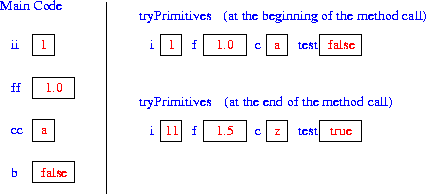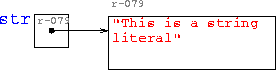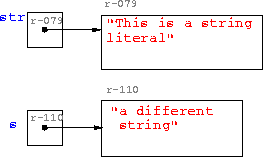University of Toronto
Department of Computer Science
csc 148: Introduction to Computer Science

Online Homework: Parameters in Java

All parameters to methods in Java are pass-by-value.

What does this mean? How do I change a parameter? This short tutorial will help you figure out how parameter passing works in Java, and will help you avoid some common mistakes.

First let's get some terminology straight. An example will help set the stage.

// Method definition
public int mult(int x, int y)
{
return x * y;
}

// Where the method mult is used
int length = 10;
int width = 5;
int area = mult(length, width);
We use the term formal parameters to refer to the parameters in the definition of the method. In the example, x and y are the formal parameters. You can remember to call them ``formal'' because they are part of the method's defintion, and you can think of a definition as being formal.

We use the term actual parameters to refer to variables in the method call, in this case length and width. They are called ``actual'' because they determine the actual values that are sent to the method.

You may have heard the term "argument" used, or just "parameter" (without specifying actual or formal). You can usually tell by the context which sort of parameter is being referred to.

What Pass-by-Value Means

Pass-by-value means that when you call a method, a copy of the value of each actual parameter is passed to the method.   You can change that copy inside the method, but this will have no effect on the actual parameter.

Unlike many other languages, Java has no mechanism for changing the value of an actual parameter. Isn't this very restrictive?  Not really. In Java, we can pass a reference to an object (also called a "handle")as a parameter. We can then change something inside the object; we just can't change what object the handle refers to. For primitives, the story is a little different.

Let's look closely at some examples of passing different kinds of things to methods: primitives, objects, strings, and array.

Passing Primitive Types

Java has eight primitive data types: six number types, character and boolean. Consider the following example:
public static void tryPrimitives(int i, double f, char c, boolean test)
{
i += 10;    //This is legal, but the new values
c = 'z';    //won't be seen outside tryPrimitives.
if(test)
test = false;
else
test = true;
f = 1.5;
}
If tryPrimitives is called within the following code, what will the final print statement produce?
int ii = 1;
double ff = 1.0;
char cc = 'a';
boolean bb = false;
// Try to change the values of these primitives:
tryPrimitives(ii, ff, cc, bb);
System.out.println("ii = " + ii + ", ff = " + ff +
", cc = " + cc + ", bb = " + bb);What's really happening here? When Java calls a method, it makes a copy of its actual parameters' values and sends the copies to the method where they become the values of the formal parameters. Then when the method returns, those copies are discarded and the actual parameters have remained unchanged.

Return values

Okay, so how do we change the values of variables inside methods? One way to do it is simply to return the value that we have changed. In the simple example below, we take two integers, a and b, as parameters and return a to the power of b.
public static int power(int a, int b)
{
int i;
int total = 1;
for(i = 0; i < b; i++)
total = total * a;
}
Now when we call the method power, number gets assigned the value that power returns.
int number = 2;
int exponent = 4;
number = power(number, exponent);
System.out.println("New value of number is " + number);
Since methods should be designed to be simple and to do one thing, you will often find that returning a value is enough.

A limitation of this approach is that you can send back only one thing from a method. What if you want to change two or more things? See the discussion of a swap method, below, for an answer.

Question

A: Given the following method:
public int tryPrimitives(int x, int y) {
x = x + 10;
y = y + 10;
return x;
}
what are the values of p, q and r after the following code executes?
int p = 1;
int q = 2;
int r = 5;
r = tryPrimitives(p, q);

Passing Object References

What if a parameter to a method is an object reference? We can manipulate the object in any way, but we cannot make the reference refer to a different object.

Suppose we have defined the following class:

class Record
{
int num;
String name;
}
Now we can pass a Record as a parameter to a method:
public static void tryObject(Record r)
{
r.num = 100;
r.name = "Fred";
}
In some other code we can create an object of our new class Record, set its fields, and call the method tryObject.
Record id = new Record();
id.num = 2;
id.name = "Barney";
tryObject(id);
System.out.println(id.name + "  " + id.num);
The print statement prints out "Fred 100"; the object has been changed in this case. Why? The reference to id is the parameter to the method, so the method cannot be used to change that reference; i.e., it can't make id reference a different Record. But the method can use the reference to perform any allowed operation on the Record that it already references.

Side Note: It is often not good programming style to change the values of instance variables outside an object. Normally, the object would have a method to set the values of its instance variables.

We cannot however make the object parameter refer to a different object by reassigning the reference or calling new on the reference. For example the following method would not work as expected:

public void createRecord(Record r, int n, String name)
{
r = new Record();
r.num = n;
r.name = name;
}
We can still encapsulate the initialization of the Record in a method, but we need to return the reference.
public Record createRecord(int n, String name)
{
Record r = new Record();
r.num = n;
r.name = name;
return r;
}

Passing Strings

When we write code with objects of class String, it can look as if strings are primitive data types. For example, when we assign a string literal to a variable, it looks no different than, say, assigning an number to an int variable. In particular, we don't have to use new.
String str = "This is a string literal.";
But in fact, a string is an object, not a primitive. A new is indeed required; it's just that Java does it behind the scenes. Java creates a new object of class String and initializes it to contain the string literal we have given it.Because str is an object, we might think that the string it contains can be changed when we pass str as a parameter to a method. Suppose we have the method tryString:

public static void tryString(String s)
{
s = "a different string";
}
When the following code is executed, what does the print statement produce?
String str = "This is a string literal.";
tryString(str);
System.out.println("str = " + str);Why is this? Maybe this picture will help you understand what goes on behind the scenes. It is important to remember what Java does when it assigns a string literal to an object. A different String object is created and the reference variable is set to point to the newly created object. In other words the value of the formal parameter, s, has changed, but this does not affect the actual parameter str. In this example, s pointed to the string object that contains "This is a string literal". After the first statement of the method executes, s points to the new string, but str has not changed.

Like other objects, when we pass a string to a method, we can in principle, change things inside the object (although we can't change which string is referenced, as we just saw). However, this capability is not useful with string because strings are "immutable". They cannot be changed because the String class does not provide any methods for modification.

Questions

B: Pass-by-value in Java means

C: Given the following method:

public void swap(int x, int y) {
int temp = x;
x = y;
y = temp;
}
what are the values of p and q after the following code executes?
int p = 20;
int q = 15;
swap(p, q);

We have to use a bit of a trick to write a swap routine that works.

Passing Arrays

Arrays are references. This means that when we pass an array as a parameter, we are passing its handle or reference. So, we can change the contents of the array inside the method.
public static void tryArray(char[] b)
{
b = 'x';
b = 'y';
b = 'z';
}
When the following code is executed, the array a does indeed have the new values in the array.
char[] a = {'a', 'b', 'c'};
tryArray(a);
System.out.println("a = " + a + ", a = " + a +
", a =" + a);
The print statements produces "a = x, a = y, a = z".

Question

D: Given the following method:
public void differentArray(float[] x)
{
x = new float;
x = 26.9f;
}
what is the output after the following code is run?
float[] xx = new float;
xx = 55.8f;
differentArray(xx);
System.out.println("xx = " + xx);

Summary

All you need to remember is that
All parameters to methods in Java are pass-by-value.

That, combined with an understanding of the difference between primitives and references, and careful tracing, will allow you to understand any Java method calls.